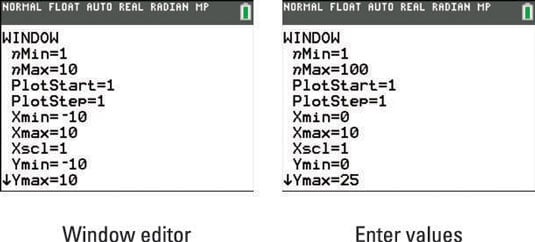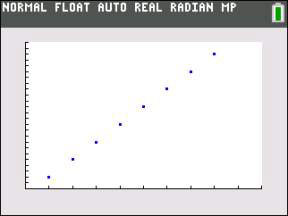##### TI-84 Plus CE Graphing Calculator For Dummies, 3rd EditionOn the TI-84 Plus calculator, it's difficult to get a nice viewing window for your sequence on your first attempt. Understanding the basics of sequence notation should help. Here are the variables used in the window of a sequence graph:

• nMin: Where n starts counting. 1 is the default value.

• nMax: Where n stops counting. Choose a value for nMax that is as large as you might need.

• PlotStart: First term number to be plotted; 1 is the default value.

• PlotStep: Increment for the n value, used only in graphing. 1 is the default value.

Remember, use the arrow keys to navigate the Window menu. Here are the steps to set your graphing window:

1. Press [WINDOW] to access the Window editor.

See the first screen.

In most cases, it is best to set nMin, PlotStart, and PlotStep to 1.2. Enter nMax.

Choose a value of nMax that is as large as you might need; try 100. See the second screen.

3. Enter the Xmin.

Enter Xmin = 0 for an aesthetically pleasing graph.

4. Enter the Xmax.

How many terms do you want to graph? Assuming the PlotStep and nMin are 1, enter the number of terms you want to graph.

5. Enter the Ymin.

Enter a value a little smaller than the smallest y-value in the sequence. Sometimes you have to guess this value; in this sequence, the smallest y-value is 2, 0 was entered in the example.

6. Enter the Ymax.

Enter a value a little larger than the largest y-value in the sequence. Sometimes you have to guess this value; in this sequence, the largest y-value was guessed to be around 25, as shown in the second screen.

If the values of Xmax or Ymax are large, you might want to adjust the Xscl or Yscl values accordingly.

Press [GRAPH]. See the graph. It looks like the Xmax needs to be larger so that all the terms in the sequence will graph.